# DATA HANDLING CLASS 7.pdf

1 de Apr de 2022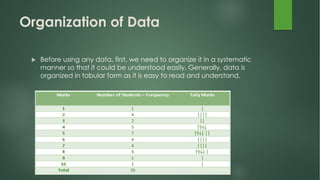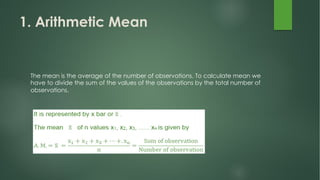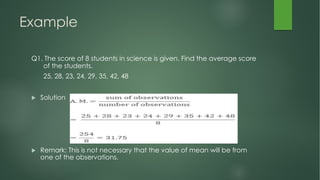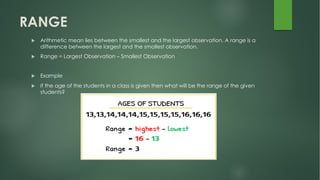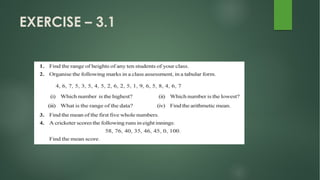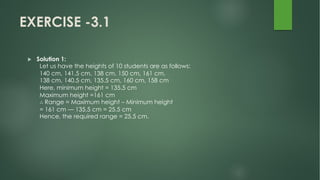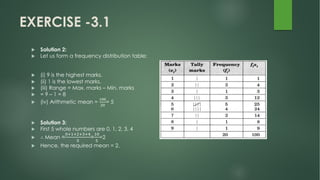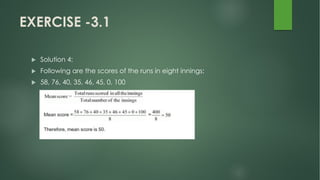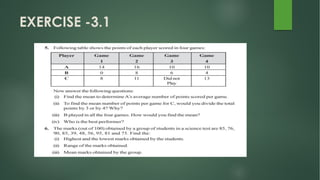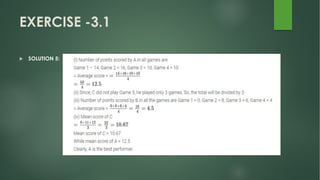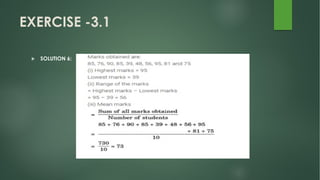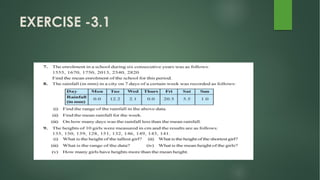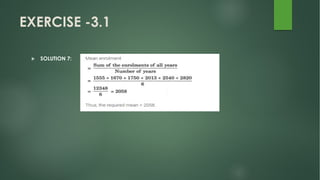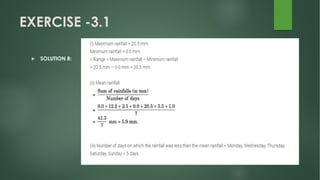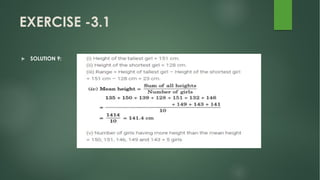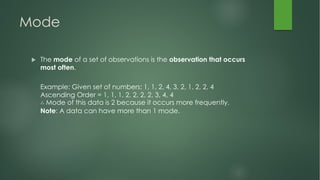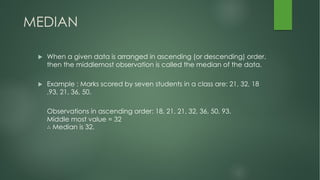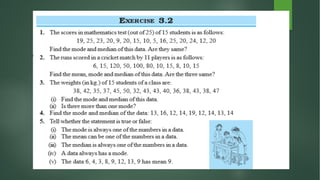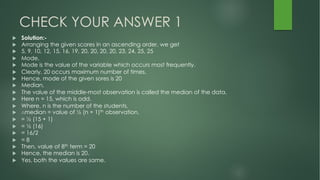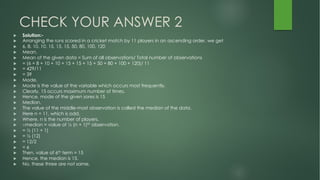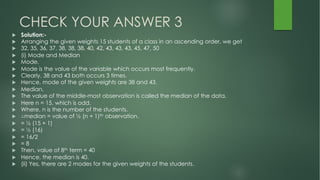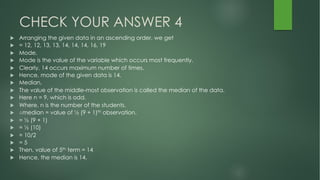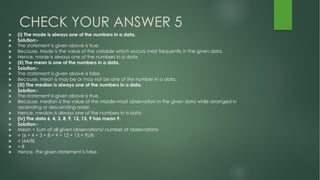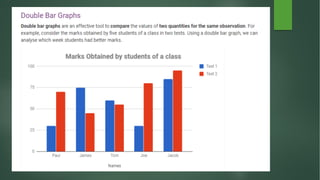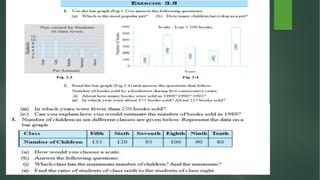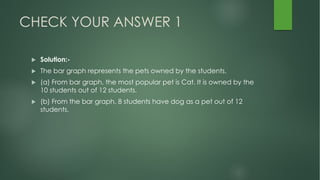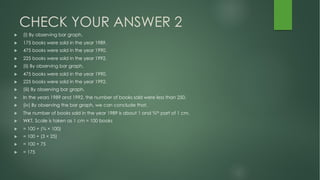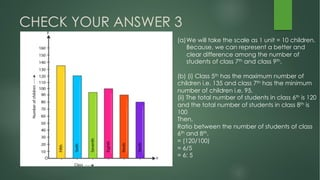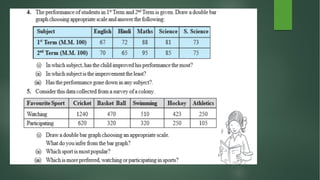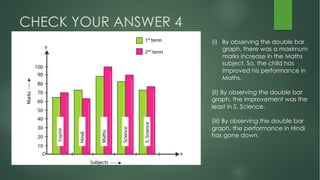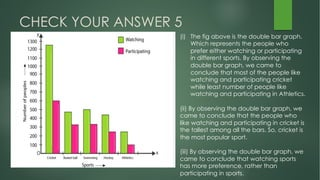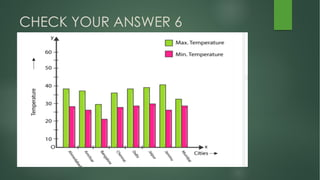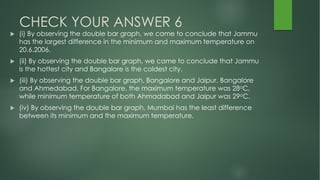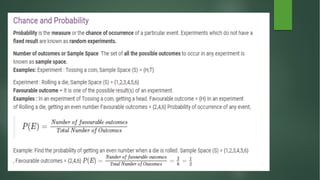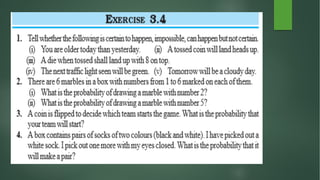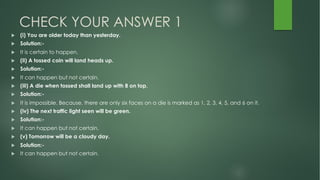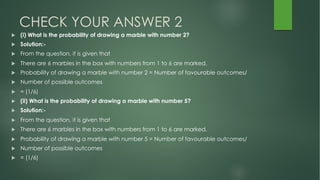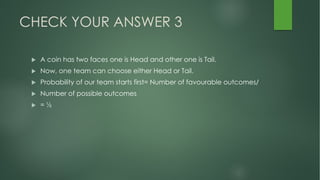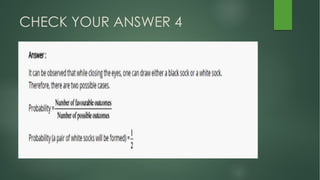1 de 44

### DATA HANDLING CLASS 7.pdf

• 2. DATA u Any raw information which we collect to know about it and to compare certain things is called Data. This information is in the form of facts and figures and is collected for some specific purpose. u Example Number of students in the class The temperature in a state on daily basis.
• 3. u Data Handling u We need to collect, organize and represent that data to draw inferences from it. This is called Data Handling. u Collecting Data u Collection of data depends upon the further requirement of the data. Before collecting any data we must know that what will be the use of data. u If we have to compare the marks of the toppers in different classes then we need the data of all the classes not only one class having any topper.
• 4. Organization of Data u Before using any data, first, we need to organize it in a systematic manner so that it could be understood easily. Generally, data is organized in tabular form as it is easy to read and understand.
• 5. Representative Values There must be a particular value which represents the complete data. This is the average of the data. The average lies between the smallest and the largest number of data so it is called Central tendency of the group of data. There are three types of central tendency of data-
• 6. 1. Arithmetic Mean The mean is the average of the number of observations. To calculate mean we have to divide the sum of the values of the observations by the total number of observations.
• 7. Example Q1. The score of 8 students in science is given. Find the average score of the students. 25, 28, 23, 24, 29, 35, 42, 48 u Solution u Remark: This is not necessary that the value of mean will be from one of the observations.
• 8. RANGE u Arithmetic mean lies between the smallest and the largest observation. A range is a difference between the largest and the smallest observation. u Range = Largest Observation – Smallest Observation u Example u If the age of the students in a class is given then what will be the range of the given students?
• 10. EXERCISE -3.1 u Solution 1: Let us have the heights of 10 students are as follows: 140 cm, 141.5 cm, 138 cm, 150 cm, 161 cm, 138 cm, 140.5 cm, 135.5 cm, 160 cm, 158 cm Here, minimum height = 135.5 cm Maximum height =161 cm ∴ Range = Maximum height – Minimum height = 161 cm — 135.5 cm = 25.5 cm Hence, the required range = 25.5 cm.
• 11. EXERCISE -3.1 u Solution 2: u Let us form a frequency distribution table: u (i) 9 is the highest marks. u (ii) 1 is the lowest marks. u (iii) Range = Max. marks – Min. marks u = 9 – 1 = 8 u (iv) Arithmetic mean = !"" #" = 5 u Solution 3: u First 5 whole numbers are 0, 1, 2, 3, 4 u ∴ Mean = !"#"\$"%"& ' = #! ' =2 u Hence, the required mean = 2.
• 12. EXERCISE -3.1 u Solution 4: u Following are the scores of the runs in eight innings: u 58, 76, 40, 35, 46, 45, 0, 100
• 20. Mode u The mode of a set of observations is the observation that occurs most often. Example: Given set of numbers: 1, 1, 2, 4, 3, 2, 1, 2, 2, 4 Ascending Order = 1, 1, 1, 2, 2, 2, 2, 3, 4, 4 ∴ Mode of this data is 2 because it occurs more frequently. Note: A data can have more than 1 mode.
• 21. MEDIAN u When a given data is arranged in ascending (or descending) order, then the middlemost observation is called the median of the data. u Example : Marks scored by seven students in a class are: 21, 32, 18 ,93, 21, 36, 50. Observations in ascending order: 18, 21, 21, 32, 36, 50, 93. Middle most value = 32 ∴ Median is 32.
• 23. CHECK YOUR ANSWER 1 u Solution:- u Arranging the given scores in an ascending order, we get u 5, 9, 10, 12, 15, 16, 19, 20, 20, 20, 20, 23, 24, 25, 25 u Mode, u Mode is the value of the variable which occurs most frequently. u Clearly, 20 occurs maximum number of times. u Hence, mode of the given sores is 20 u Median, u The value of the middle-most observation is called the median of the data. u Here n = 15, which is odd. u Where, n is the number of the students. u ∴median = value of ½ (n + 1)th observation. u = ½ (15 + 1) u = ½ (16) u = 16/2 u = 8 u Then, value of 8th term = 20 u Hence, the median is 20. u Yes, both the values are same.
• 24. CHECK YOUR ANSWER 2 u Solution:- u Arranging the runs scored in a cricket match by 11 players in an ascending order, we get u 6, 8, 10, 10, 15, 15, 15, 50, 80, 100, 120 u Mean, u Mean of the given data = Sum of all observations/ Total number of observations u = (6 + 8 + 10 + 10 + 15 + 15 + 15 + 50 + 80 + 100 + 120)/ 11 u = 429/11 u = 39 u Mode, u Mode is the value of the variable which occurs most frequently. u Clearly, 15 occurs maximum number of times. u Hence, mode of the given sores is 15 u Median, u The value of the middle-most observation is called the median of the data. u Here n = 11, which is odd. u Where, n is the number of players. u ∴median = value of ½ (n + 1)th observation. u = ½ (11 + 1) u = ½ (12) u = 12/2 u = 6 u Then, value of 6th term = 15 u Hence, the median is 15. u No, these three are not same.
• 25. CHECK YOUR ANSWER 3 u Solution:- u Arranging the given weights 15 students of a class in an ascending order, we get u 32, 35, 36, 37, 38, 38, 38, 40, 42, 43, 43, 43, 45, 47, 50 u (i) Mode and Median u Mode, u Mode is the value of the variable which occurs most frequently. u Clearly, 38 and 43 both occurs 3 times. u Hence, mode of the given weights are 38 and 43. u Median, u The value of the middle-most observation is called the median of the data. u Here n = 15, which is odd. u Where, n is the number of the students. u ∴median = value of ½ (n + 1)th observation. u = ½ (15 + 1) u = ½ (16) u = 16/2 u = 8 u Then, value of 8th term = 40 u Hence, the median is 40. u (ii) Yes, there are 2 modes for the given weights of the students.
• 26. CHECK YOUR ANSWER 4 u Arranging the given data in an ascending order, we get u = 12, 12, 13, 13, 14, 14, 14, 16, 19 u Mode, u Mode is the value of the variable which occurs most frequently. u Clearly, 14 occurs maximum number of times. u Hence, mode of the given data is 14. u Median, u The value of the middle-most observation is called the median of the data. u Here n = 9, which is odd. u Where, n is the number of the students. u ∴median = value of ½ (9 + 1)th observation. u = ½ (9 + 1) u = ½ (10) u = 10/2 u = 5 u Then, value of 5th term = 14 u Hence, the median is 14.
• 27. CHECK YOUR ANSWER 5 u (i) The mode is always one of the numbers in a data. u Solution:- u The statement is given above is true. u Because, Mode is the value of the variable which occurs most frequently in the given data. u Hence, mode is always one of the numbers in a data. u (ii) The mean is one of the numbers in a data. u Solution:- u The statement is given above is false. u Because, mean is may be or may not be one of the number in a data. u (iii) The median is always one of the numbers in a data. u Solution:- u The statement is given above is true. u Because, median is the value of the middle-most observation in the given data while arranged in ascending or descending order. u Hence, median is always one of the numbers in a data u (iv) The data 6, 4, 3, 8, 9, 12, 13, 9 has mean 9. u Solution:- u Mean = Sum of all given observations/ number of observations u = (6 + 4 + 3 + 8 + 9 + 12 + 13 + 9)/8 u = (64/8) u = 8 u Hence, the given statement is false.
• 30. CHECK YOUR ANSWER 1 u Solution:- u The bar graph represents the pets owned by the students. u (a) From bar graph, the most popular pet is Cat. It is owned by the 10 students out of 12 students. u (b) From the bar graph, 8 students have dog as a pet out of 12 students.
• 31. CHECK YOUR ANSWER 2 u (i) By observing bar graph, u 175 books were sold in the year 1989. u 475 books were sold in the year 1990. u 225 books were sold in the year 1992. u (ii) By observing bar graph, u 475 books were sold in the year 1990. u 225 books were sold in the year 1992. u (iii) By observing bar graph, u In the years 1989 and 1992, the number of books sold were less than 250. u (iv) By observing the bar graph, we can conclude that, u The number of books sold in the year 1989 is about 1 and ¾th part of 1 cm. u WKT, Scale is taken as 1 cm = 100 books u = 100 + (¾ × 100) u = 100 + (3 × 25) u = 100 + 75 u = 175
• 32. CHECK YOUR ANSWER 3 (a)We will take the scale as 1 unit = 10 children. Because, we can represent a better and clear difference among the number of students of class 7th and class 9th. (b) (i) Class 5th has the maximum number of children i.e. 135 and class 7th has the minimum number of children i.e. 95. (ii) The total number of students in class 6th is 120 and the total number of students in class 8th is 100 Then, Ratio between the number of students of class 6th and 8th, = (120/100) = 6/5 = 6: 5
• 34. CHECK YOUR ANSWER 4 (i) By observing the double bar graph, there was a maximum marks increase in the Maths subject. So, the child has improved his performance in Maths. (ii) By observing the double bar graph, the improvement was the least in S. Science. (iii) By observing the double bar graph, the performance in Hindi has gone down.
• 35. CHECK YOUR ANSWER 5 (i) The fig above is the double bar graph. Which represents the people who prefer either watching or participating in different sports. By observing the double bar graph, we came to conclude that most of the people like watching and participating cricket while least number of people like watching and participating in Athletics. (ii) By observing the double bar graph, we came to conclude that the people who like watching and participating in cricket is the tallest among all the bars. So, cricket is the most popular sport. (iii) By observing the double bar graph, we came to conclude that watching sports has more preference, rather than participating in sports.
• 36. u 6. Take the data giving the minimum and the maximum temperature of various cities given in the beginning of this chapter (Table 3.1). Plot a double bar graph using the data and answer the following: u (i) Which city has the largest difference in the minimum and maximum temperature on the given date? u (ii) Which is the hottest city and which is the coldest city? u (iii) Name two cities where maximum temperature of one was less than the minimum temperature of the other. u (iv) Name the city which has the least difference between its minimum and the maximum temperature.
• 38. CHECK YOUR ANSWER 6 u (i) By observing the double bar graph, we came to conclude that Jammu has the largest difference in the minimum and maximum temperature on 20.6.2006. u (ii) By observing the double bar graph, we came to conclude that Jammu is the hottest city and Bangalore is the coldest city. u (iii) By observing the double bar graph, Bangalore and Jaipur, Bangalore and Ahmedabad. For Bangalore, the maximum temperature was 28oC, while minimum temperature of both Ahmadabad and Jaipur was 29oC. u (iv) By observing the double bar graph, Mumbai has the least difference between its minimum and the maximum temperature.
• 41. CHECK YOUR ANSWER 1 u (i) You are older today than yesterday. u Solution:- u It is certain to happen. u (ii) A tossed coin will land heads up. u Solution:- u It can happen but not certain. u (iii) A die when tossed shall land up with 8 on top. u Solution:- u It is impossible. Because, there are only six faces on a die is marked as 1, 2, 3, 4, 5, and 6 on it. u (iv) The next traffic light seen will be green. u Solution:- u It can happen but not certain. u (v) Tomorrow will be a cloudy day. u Solution:- u It can happen but not certain.
• 42. CHECK YOUR ANSWER 2 u (i) What is the probability of drawing a marble with number 2? u Solution:- u From the question, it is given that u There are 6 marbles in the box with numbers from 1 to 6 are marked. u Probability of drawing a marble with number 2 = Number of favourable outcomes/ u Number of possible outcomes u = (1/6) u (ii) What is the probability of drawing a marble with number 5? u Solution:- u From the question, it is given that u There are 6 marbles in the box with numbers from 1 to 6 are marked. u Probability of drawing a marble with number 5 = Number of favourable outcomes/ u Number of possible outcomes u = (1/6)
• 43. CHECK YOUR ANSWER 3 u A coin has two faces one is Head and other one is Tail. u Now, one team can choose either Head or Tail. u Probability of our team starts first= Number of favourable outcomes/ u Number of possible outcomes u = ½